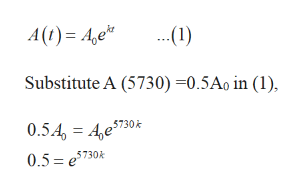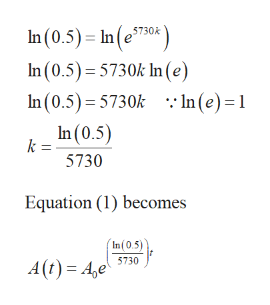# Dinosaur fossils are too old to be reliably dated using carbon-14, which has a half-life of about 5730 years. Suppose we had a 66 million year old dinosaur fossil. How much of the living dinosaur's 14C would be remaining today? (Round your answer to five decimal places.) % Suppose the minimum detectable amount is 0.5%. What is the maximum age of a fossil that we could date using 14C? (Round your answer to the nearest integer.) yr

Question
31 views
Dinosaur fossils are too old to be reliably dated using carbon-14, which has a half-life of about 5730 years. Suppose we had a 66 million year old dinosaur fossil. How much of the living dinosaur's 14C would be remaining today? (Round your answer to five decimal places.)
%

Suppose the minimum detectable amount is 0.5%. What is the maximum age of a fossil that we could date using 14C? (Round your answer to the nearest integer.)
yr

check_circle

Step 1

a)

Given,

The half life 14C is 5730 years.

Step 2

The formula for exponential model ishelp_outlineImage Transcriptionclose..(1) A(t) = A,e* Substitute A (5730) =0.5Ao in (1), 0.5.4, = 4,e\$730k 0.5 = e 5730k fullscreen
Step 3

Taking ln on both sides,

...help_outlineImage TranscriptioncloseIn (0.5) = In(e50 ) 5730k In (0.5) = 5730k In (e) In (0.5)= 5730k :: In(e)=1 In (0.5) k : 5730 Equation (1) becomes In (0.5) 5730 A(t) = A,e fullscreen

### Want to see the full answer?

See Solution

#### Want to see this answer and more?

Solutions are written by subject experts who are available 24/7. Questions are typically answered within 1 hour.*

See Solution
*Response times may vary by subject and question.
Tagged in

### Other